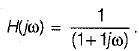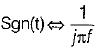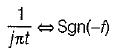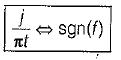Courses

# Continuous Time Fourier Transformer (CTFT) - 1

## 10 Questions MCQ Test Topicwise Question Bank for GATE Electrical Engineering | Continuous Time Fourier Transformer (CTFT) - 1

Description
This mock test of Continuous Time Fourier Transformer (CTFT) - 1 for Electronics and Communication Engineering (ECE) helps you for every Electronics and Communication Engineering (ECE) entrance exam. This contains 10 Multiple Choice Questions for Electronics and Communication Engineering (ECE) Continuous Time Fourier Transformer (CTFT) - 1 (mcq) to study with solutions a complete question bank. The solved questions answers in this Continuous Time Fourier Transformer (CTFT) - 1 quiz give you a good mix of easy questions and tough questions. Electronics and Communication Engineering (ECE) students definitely take this Continuous Time Fourier Transformer (CTFT) - 1 exercise for a better result in the exam. You can find other Continuous Time Fourier Transformer (CTFT) - 1 extra questions, long questions & short questions for Electronics and Communication Engineering (ECE) on EduRev as well by searching above.
QUESTION: 1

Solution:
QUESTION: 2

Solution:
QUESTION: 3

### The fourier transform of real value time signal has

Solution:
QUESTION: 4

The signal x(t) is a real and odd function of ‘t’ the x(ω) is

Solution:
QUESTION: 5

A linear system has the transfer functionWhen it is subjected to an input white noise process with a constant spectral dencity ‘A’ the spectral density of the output will be

Solution:
QUESTION: 6

The auto -correlation of a wide sense stationary random process is given by e-2|τ|. The peak valve of the spectral density is

Solution:
QUESTION: 7

Which one of the following is the correct fourier transform of the unit step signal?
u(t) = 1 for t > 0
= 0 for t < 0

Solution:
QUESTION: 8

The fourier transform of a conjugate symmetric function is always

Solution:
QUESTION: 9

The inverse fourier transform of sgn(f)

Solution:then∴∴QUESTION: 10

Fourier transform f(jω) of an arbitrary signal has the property

Solution: